# tkinter的简单对话框，不简单

### 导入简单对话框模块

`from tkinter import simpledialog # 简单对话框模块`

### 自定义对话框

```from tkinter import *
from tkinter import simpledialog # 简单对话框模块

root=Tk()
root.title('测试')
a=simpledialog.SimpleDialog(root,title='请选择',text='选择喜欢的三国人物',
buttons=['曹操','刘备','孙权','诸葛亮','关羽','张飞','赵云'],) # 弹出对话框

b=['曹操','刘备','孙权','诸葛亮','关羽','张飞','赵云']

a.go()  # 先执行go()，下面的num方法才起作用
print(a.num) # 输出按钮的序号
print(b[a.num])   # 输出按钮的文本

root.mainloop()```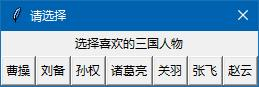```from tkinter import *
from tkinter import simpledialog # 简单对话框模块

root=Tk()
root.title('测试')
a=simpledialog.SimpleDialog(root,title='请选择',text='选择喜欢的三国人物',
buttons=['曹操','刘备','孙权','诸葛亮','关羽','张飞','赵云'],
cancel=-1) # 弹出对话框

b=['曹操','刘备','孙权','诸葛亮','关羽','张飞','赵云']

a.go()  # 先执行go()，下面的num方法才起作用
print(a.num) # 输出按钮的序号

print("对话框被关闭") if a.num == -1 else print(b[a.num])

root.mainloop()```

```from tkinter import *
from tkinter import simpledialog # 简单对话框模块

def popup():
print(a)  # 输出返回值

root=Tk()
but1=Button(root,text=" 弹出 ",command=popup)
but1.pack()

root.mainloop()```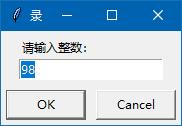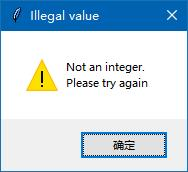```from tkinter import *

from tkinter import simpledialog # 简单对话框模块

def popup():

print(a) # 输出返回值

root=Tk()

but1=Button(root,text=" 弹出 ",command=popup)

but1.pack()

root.mainloop()```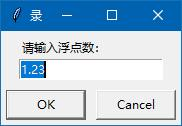如果你会举一反三，那字符串对话框就很好理解了，这个字符串对话框跟上述的2个对话框在语法上一致的。

```from tkinter import *
from tkinter import simpledialog # 简单对话框模块

def popup():
print(a) # 输出返回值

root=Tk()
but1=Button(root,text=" 弹出 ",command=popup)
but1.pack()

root.mainloop()```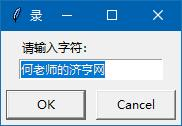<< 上一篇 下一篇 >>